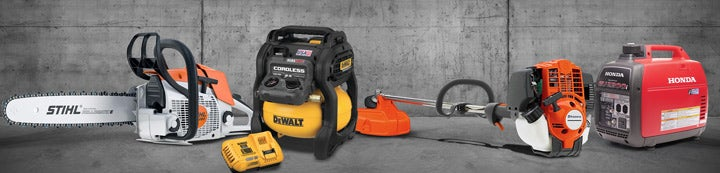1 - 1 of 1 Posts

#### bemegopi

·
##### Registered
Joined
·
3 Posts
Discussion Starter · ·
If i know the double acting cylinder CFM? what are the uses for calculating the double acting cylinder CFM??

It is the correct method??

1.Find the area of the piston by converting the bore diameter into square inches.
(2 in. bore/2)2 x 3.1416 (Π) = 3.14 sq. in.

2. Determine consumption per single stroke.
3.14 sq. in. x 4 in. stroke = 12.56 cu.in.

3. Determine consumption per complete cycle (Disregard displacement of piston rod because
it is generally not significant).

12.56 cu.in. x 2 = 25.12 cu.in. per cycle
4. Determine volume of 80 PSI air that is consumed per minute.
25.12 cu.in. x 30 cycles/minute = 753.6 cu.in./min. of 80 PSI air

5. Convert cu.in. to cu.ft.
753.6 cu.in./min. = 0.436 cu.ft./min.
1728 cu.in/cu.ft.

6. Convert air compressed to 80 PSI to “free” (uncompressed) air.
80 PSI + 14.7 PSI = 6.44 (times air is compressed when at 80 PSI)
14.7 PSI

7. Determine cubic feet of free air used per minute.
0.436 cu. ft. x 6.44 compression ratio = 2.81 cu. ft. of free air used per minute

8. So, the consumption rate of a 2″ bore, 4″ stroke cylinder operating 30 complete cycles per
minute at 80 PSI is 2.81 SCFM (Standard Cubic Feet Per Minute) of free air. “Standard”
means at a temperature of 70°F and at sea level. Also see questions regarding Cv (pg. 1) and cylinder
size selection

And how to convert SCFM to CFM???

1 - 1 of 1 Posts